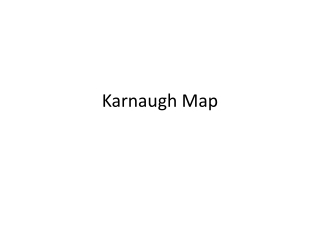DownloadDownload PresentationKarnaugh Map

# Karnaugh Map

Download Presentation## Karnaugh Map

- - - - - - - - - - - - - - - - - - - - - - - - - - - E N D - - - - - - - - - - - - - - - - - - - - - - - - - - -
##### Presentation Transcript

1. Karnaugh Map

2. Simplifying Digital Circuits • Reconsider the 1-bit full adder. The carry bit is • But we can implement the function with a much simpler circuit: • How to get there?

3. Simplifying digital circuit • There are many methods. • Using boolean algebra • Using K-map • By just being really smart…

4. Boolean Algebra Laws A+A=A, A*A=A

5. Boolean Algebra • To use Boolean algebra, note that CO= abc’+ab’c+a’bc+abc • Now, • abc’+abc=ab(c’+c)=ab. • ab’c+abc=ac(b’+b)=ac • a’bc+abc=bc(a’+a)=bc • We used term abc three times because abc=abc+abc+abc!

6. K-map • It is actually more convenient to use K-map to simplify digital circuits. • K-map is a very mechanical procedure. Nothing fancy. • It basically uses two rules: A+A=A, and AB+AB’=A.

7. K-map • K-map • CO = ab + ac + bc ab c 00 01 10 11 0 1

8. K-map rules • Draw the K-map. Remember to make sure that the adjacent rows/columns differ by only one bit. • According to the truth table, write 1 in the boxes. • Draw a circle around a rectangle with all 1s. The rectangle must have size 2,4,8,16…Then, reduce the term by writing down the variables that the values does not change. For example, if there is a rectangle with two 1s representing abc’ and abc, you write a term as ab. • A term may be covered in multiple circles. • The rectangle can wrap-around!

9. K-map • F=a’bc’+a’bc+a’b’c+ab’c ab c 00 01 10 11 0 1

10. K-map • F=a’bc’+a’bc+a’b’c+ab’c • F=a’c+a’b+b’c ab c 00 01 10 11 0 1

11. K-map • F=a’bc’+a’bc+abc’+abc+a’b’c ab c 00 01 10 11 0 1

12. K-map • F=a’bc’+a’bc+abc’+abc+a’b’c • F=b+a’c ab c 00 01 10 11 0 1

13. K-map • F=a’bc’d+a’bcd+abc’d+abcd+a’b’c’d+abcd’ ab cd 00 01 10 11 00 01 11 10

14. K-map • F=a’bc’d+a’bcd+abc’d+abcd+a’b’c’d+abcd’ • F=bd+a’c’d+abc ab cd 00 01 10 11 00 01 11 10

15. Design a selector? • I need a circuit that takes two input bits, a and b, and a selector bit s. The function is that if s=0, f=a. if s=1, f=b.

16. Selector

17. K-map • F=s’ab’+s’ab+sa’b+sab sa b 00 01 10 11 0 1

18. K-map • F=s’ab’+s’ab+sa’b+sab • F=s’a+ab sa b 00 01 10 11 0 1

19. In MIPS processor